# A spring-mass system is subjected to a sinusoidal force with a frequency of 5 rad/s. The force transmissibility is 1.35. If the frequency is increased slightly, the force transmissibility decreases. The spring?s stiffness is 1000 N/m. The system has negligible (but non-zero) damping. What is the mass of the system?

Question
1 views

A spring-mass system is subjected to a sinusoidal force with a frequency of 5 rad/s. The force transmissibility is 1.35. If the frequency is increased slightly, the force transmissibility decreases. The spring?s stiffness is 1000 N/m. The system has negligible (but non-zero) damping. What is the mass of the system?

check_circle

Step 1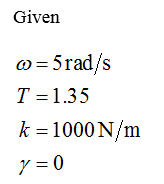Step 2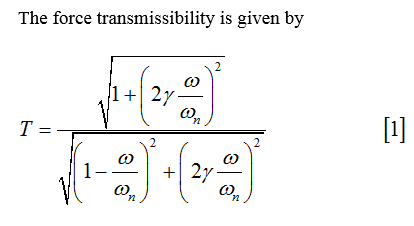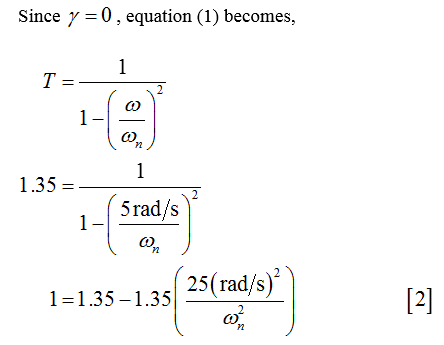Step 3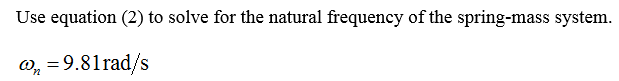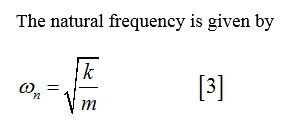...

### Want to see the full answer?

See Solution

#### Want to see this answer and more?

Solutions are written by subject experts who are available 24/7. Questions are typically answered within 1 hour.*

See Solution
*Response times may vary by subject and question.
Tagged in